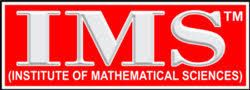# Analysis of IFoS Mathematics Paper -I & II

IFoS Mathematics examination more or less following pattern of examination is going on: Question Paper Model (from 2011). Candidates should attempt questions 1 and 5 which are compulsory , any three of the remaining questions selecting atleast one question from each section.

### Question Paper Model (from 2011) Paper – I

Time-3hours
Max Marks : 200

 Q1. a, b LA 10×4=40 c, d CAL e 3-D Q2. a, b, c, d LA 10×4=40 Q3. a, b, c ,d CAL 10×4=40 Q4. a, b ,c ,d 3-D 10×4=40

### SECTION – B

 Q5. a, b ODE 10×4=40 c, d Dy & Statics & Hydrostatics e, f VA Q6. a, b, c, d ODE 10×4=40 Q7. a, b, c Dynamics, Statics and Hydro Statics 14 + 13 + 13= 40 Q8. a, b, c, d VA 10 × 4 = 40

## IFoS : Paper-II Question Paper Model (from 2011)

Candidates should attempt questions 1 and 5 which are compulsory , any three of the remaining questions  electing atleast one question from each section.

Time : 3 Hours
Max Marks : 200

### SECTION – A

 Q1. a, b MA 10 × 4 = 40 c, d RA e CA & LPP Q2. a, b, c MA RA CA 13 13 14 = 40 Q3. a, b, c RA MA LPP 13 13 14 = 40 Q4. a, b, c RA CA LPP 13 13 14 = 40

### Section-B

 Q5. a PDE 10 × 4 = 40 b, c NA d, e Mech & Fluid & Dy Q6. a PDE 16 12 12 = 40 b, c NA & CP Q7. a, b, c Fluid Dy PDE NA 16 12 12 = 40 Q8. a, b, c Mech CP Fluid Dy 14 12 12 = 40

### LEGEND

• LA : Linear Algebra
• CAL : Calculus
• 3-D : 3-Dimensional Geometry
• ODE : Ordinary Differential Equations
• Dy & Statics : Dynamics & Statics
• VA : Vector Analysis
• MA : Modern Algebra
• RA : Real Analysis
• CA : Complex Analysis
• LPP : Linear Programming Problem
• PDE : Partial Differential Equations
• NA & CP : Numerical Analysis and Computer Programming
• Mech & Fluid Dy : Mechanics & Fluid Dynamics